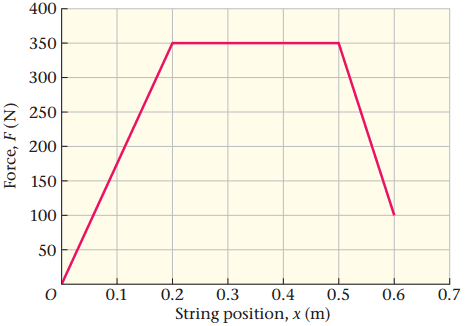# Work problem: force vs distance curve for compound bow

## Homework Statement

A compound bow in archery allows the user to hold the bowstring at full draw with considerably less force than the maximum force exerted by the string. The draw force as a function of the string position x for a particular compound bow is shown in (Figure 1) .

Part A
How much work does the archer do on the bow in order to draw the string from x=0 to x=0.60m?## Homework Equations

area= work

total work=sum of all areas

## The Attempt at a Solution

(.2m x 350 N)/2 + (.3m x 350 N) + (.1m x 250 N)/2 = 152.5J

153J for three sig figs.[/B]

Apparently the answer is wrong, but I do not know what I did wrong? I found the area of the two triangles, and the rectangle in the three equations above.

phinds
Gold Member
But the area is NOT "two triangles and a rectangle". Do you see why I say that?

But the area is NOT "two triangles and a rectangle". Do you see why I say that?
No I do not. Is it because from .5m to .6m it is not a complete triangle?

gneill
Mentor
Your thread title is too general. I've modified it to better identify the type of problem.

You've missed a bit of the area under the curve. Take a closer look at the region of the smaller triangle: what's it "sitting" on?

edit: Ah. Phinds got in there before me!

Your thread title is too general. I've modified it to better identify the type of problem.

You've missed a bit of the area under the curve. Take a closer look at the region of the smaller triangle: what's it "sitting" on?

edit: Ah. Phinds got in there before me!

the smaller triangle is sitting on a small rectangle between .5m to .6m?

gneill
Mentor
the smaller triangle is sitting on a small rectangle between .5m to .6m?
Right.

the smaller triangle is sitting on a small rectangle between .5m to .6m?

That was tricky, but I got it now. Thanks!!